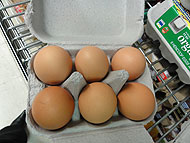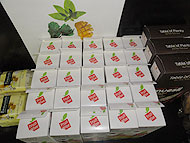# Visualising arrays

An array is a rectangular arrangement of objects in equal rows (horizontal) and equal columns (vertical).

Everyday examples of arrays include a muffin tray and an egg carton.An array of eggs.An array of juice boxes.An array of chocolates.

Interpreting the structure of arrays requires spatial visualisation processes.

Students need to connect objects together and treat them as a whole collection.

When constructing an array, students combine both spatial (rows of squares) and numeric composites (number of squares in a row).

When part of the array is screened, students have to visualise the composite units.

Recognising the unit of repeat – individual rows or columns – is important.

In a rectangular grid showing 3 rows of 4, the unit of repeat can be three. This can lead to skip counting by 4 to find the total number of 12 squares. Alternatively, the unit of repeat can be four, leading to skip counting by 3 to find the total number of 12 squares.

Students can generalise that 3 rows of 4 columns, or 4 rows of 3 columns, show that 3 $$\times$$ 4 = 4 $$\times$$ 3, which is the commutative property.

Knowing the commutative facts reduces the number of multiplication facts students need to remember.

The rectangular array structure gives a visual pattern to both multiplication and division.

For example, 6 rows of 4 is 24 can be interpreted as 24 divided by 6 is 4.

## What do you see?

The structure of a rectangular array assists students to visualise the multiplicative situation. The group is seen as a composite unit rather than as a collection of individual items.

## Partial arrays

Array structures are ways to represent factors. In this activity, students are given the number of tiles in each row and column and are asked to find the total number of tiles needed to construct the rectangle.